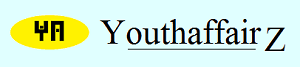May 2012

# Solution

Let us number the coins as 1, 2, 3, 4, 5, 6, 7, 8, 9, 10, 11 and 12.

Let us divide the twelve coins into three groups, each containing four coins. Therefore, Group I has coins 1, 2, 3 and 4. Group II has coins 5, 6, 7 and 8 and Group III has coins 9, 10, 11 and 12.

Let us weigh Group I against Group II. This will be the first weighing.

Now, either the two groups will balance or they will not.

First let us take the situation where they balance: Since the two groups have balanced, this means the coins in both these groups are genuine and the fake coin is in Group III. Take any three genuine coins (from the first two groups) and weigh them (this is the second weighing) against three coins in Group III. If they balance, the fourth coin in Group III is the fake coin. But, we do not know whether it is heavier or lighter. So a third weighing, wherein the fake coin is weighed against a real coin will determine this. If the three genuine coins do not balance against the three coins from Group III, then one of the three Group III coins is fake. But we can also state for sure now whether the fake coin is lighter or heavier (the fake coin is one of the three Group III coins and if while weighing them against the genuine coins, the Group III side dips, the fake coin is heavier else it is light.) One last weighing is left, so weigh two coins from the three Group III coins. if they balance, the third coin is fake, else the the coin which is heavier or lighter (as determined by the second weighing) is fake.

Now let us go back to the first weighing and consider the case when Group I coins do not balance against Group II coins. So the fake coin is in one of these two groups. Let us mark the group that was heavier as "Group A" and the group that was lighter as "Group B". For the second weighing, take two coins from "Group A" and one coin from "Group B" and weigh them against the other two coins of "Group A" and one coin from "Group B". If they balance, the fake coin must be one of the two remaining "Group B" coins. A weighing between these two coins will tell us which is the fake coin. The lighter coin will be the fake one as "Group B" is lighter. However, if the two coins from "Group A" and one coin from "Group B" and the other two coins from "Group A" and one coin from "Group B" did not balance, then look at the heavier side. Either the fake coin is one of the two "Group A" coins or it can the "Group B" coin on the other side of the scale. Take the two "Group A" coins and weigh them against each other. If they balance, then it is the "Group B" coin that is fake, and if they don't balance, then the heavier one is the fake coin.

• Union Public Service Commission - www.upsc.gov.in
• IIT-Kharagpur - www.iitkgp.ac.in
• Indian Statistical Institute - www.isical.ac.in
• Indian Institute of Technology Madras - www.iitm.ac.in
• Indian Institute of Management, Ahmedabad - www.iimahd.ernet.in
• Indian Institute of Mass Commission - www.iimc.nic.in
• IIT Bombay - www.iitb.ac.in